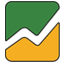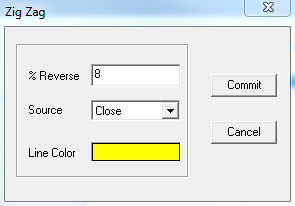# Zig Zag

User can add Zig Zag indicator to their chart by right click the chart and select Study Config to open the study configure window first, then select the “Zig Zag” from the study list and add it into the Studies In Chart field.Zig Zag Configure window allows user to modify the Zig Zag chart, such as change the study percentage and line’s color. User can open this Zig Zag Configure window either by click the “Config” button or double click the study “Zig Zag” under Studies In Chart section

## Explanation of Each Field

·         % Reverse: Allows user to adjust reverse percentage for the Zig Zag study.

·         Source: Use the dropdown menu to select the study source for the Zig Zag study.

Ø  Open: Use the open price data to calculate the Zig Zag.

Ø  High: Use the highest price data to calculate the Zig Zag.

Ø  Low: Use the lowest price data to calculate the Zig Zag.

Ø  Close: Use the close price data to calculate the Zig Zag.

Ø  (H+L)/2: Use this equation to calculate the price first, then use those price data to calculate the Zig Zag.

Ø  (H+L+C)/3: Use this equation to calculate the price first, then use those price data to calculate the Zig Zag.

Ø  (O+H+L+C)/4: Use this equation to calculate the price first, then use those price data to calculate the Zig Zag.

Ø  (H+L+C+C)/4: Use this equation to calculate the price first, then use those price data to calculate the Zig Zag.

(Hint, O means Open Price, H means Highest Price, L means Lowest Price, and C means Close Price. And in (H+L+C+C)/4, both Cs are refer to Close Price, so same as (H+L+2C)/4.)

·         Line Color: Click on the color field allows user to change the line color.